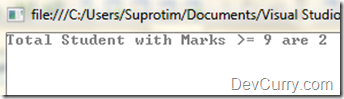Simple Count based on a Condition using LINQ

 Tweet

Here’ a simple example demonstrating how to perform a count on a List<> based on a condition

C#

`class Program{    static void Main(string[] args)    {        List<Students> students = new List<Students>();        students.Add(new Students() { StudentId = 1, Marks = 8.0f });        students.Add(new Students() { StudentId = 2, Marks = 5.0f });        students.Add(new Students() { StudentId = 3, Marks = 7.0f });        students.Add(new Students() { StudentId = 4, Marks = 9.5f });        students.Add(new Students() { StudentId = 1, Marks = 9.0f });        var topStudCount = students.Count(r => r.Marks >= 9.0f);        Console.WriteLine("Students with Marks >=9 are {0}", topStudCount);        Console.ReadLine();    }}class Students{    public int StudentId { get; set; }    public float Marks { get; set; }}`

VB.NET

Use this Converter Tool

OUTPUT